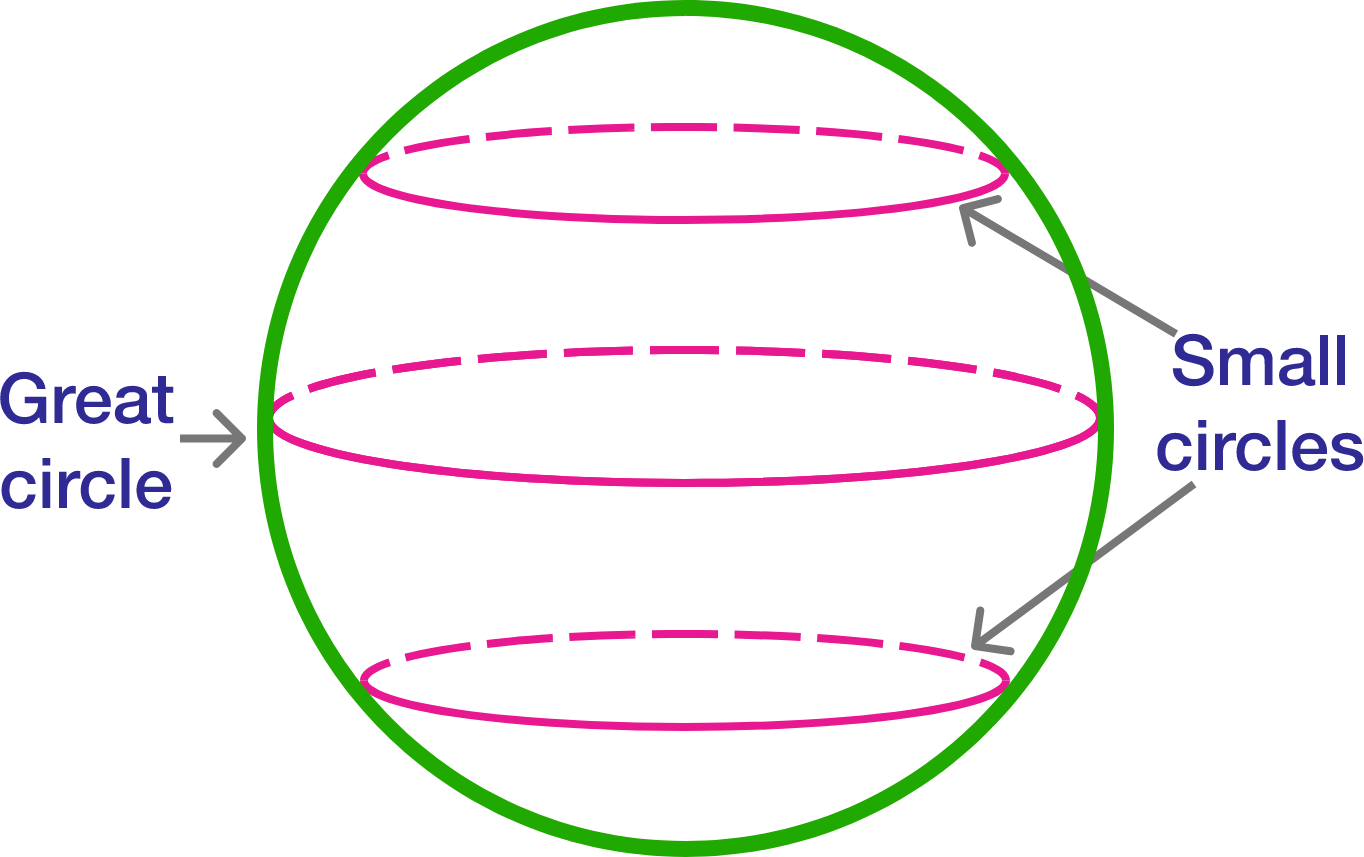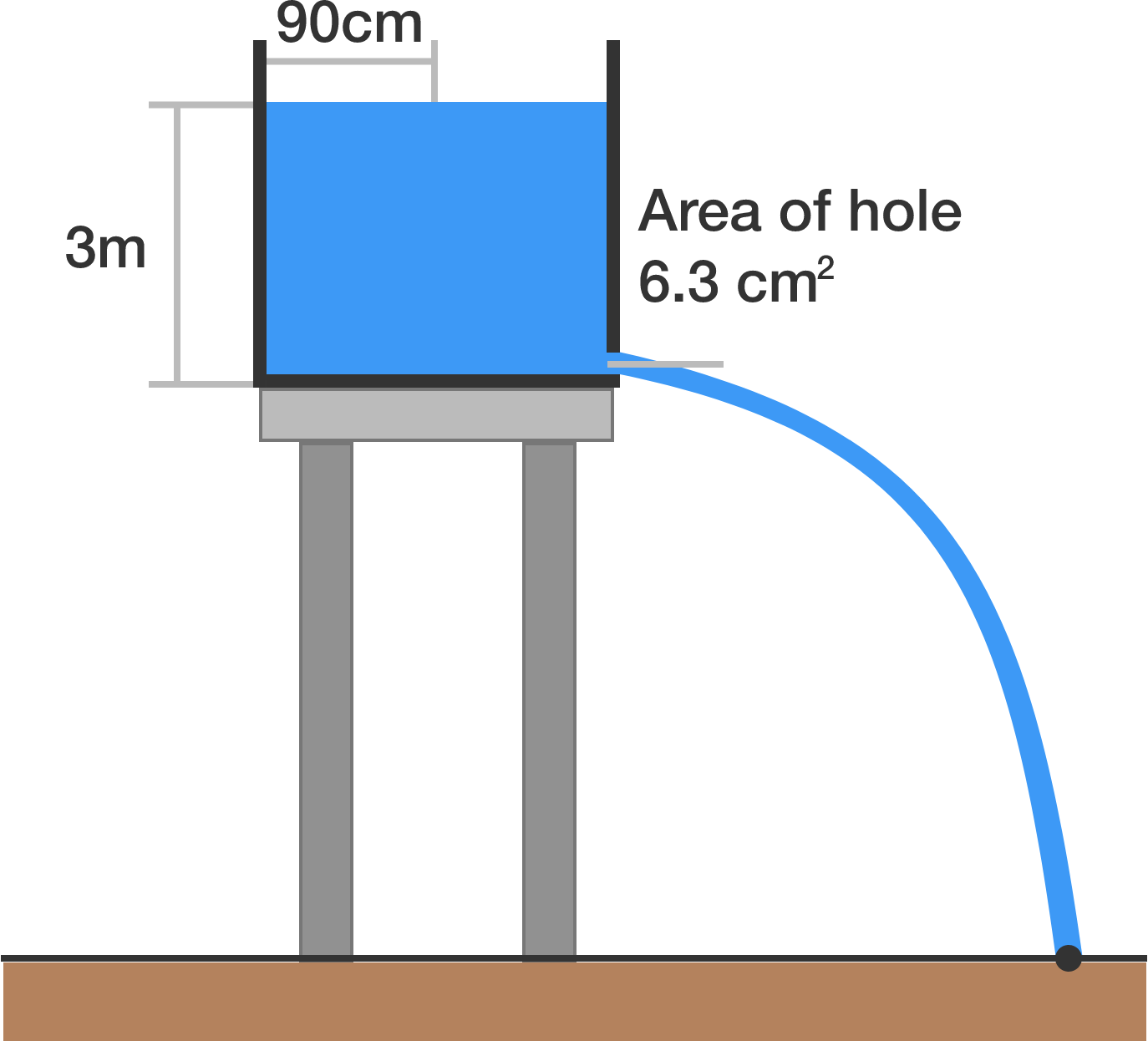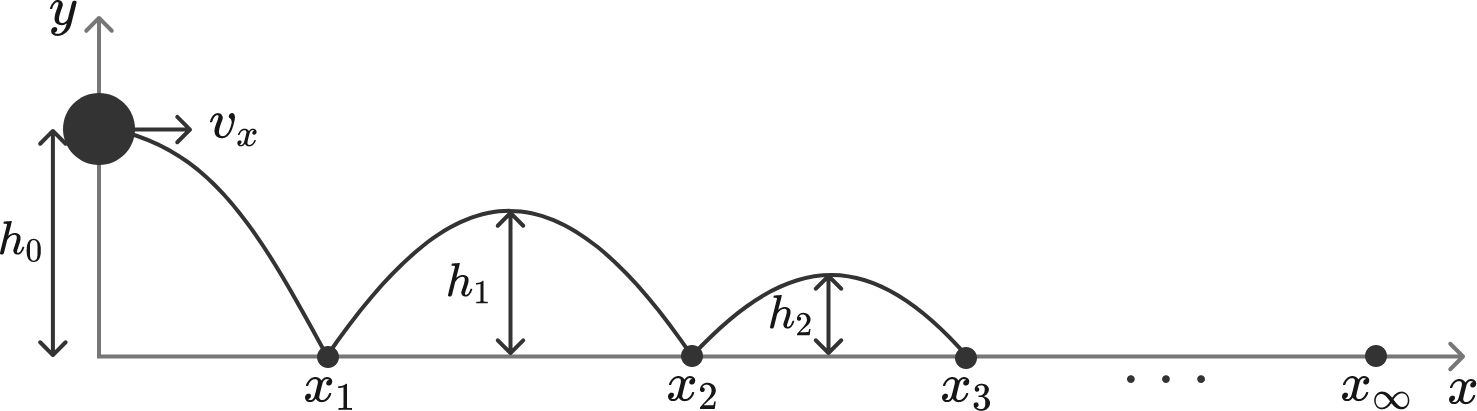# Problems of the Week

Contribute a problem

Given a sphere, a circle is called a great circle if it is the intersection of the sphere with a plane passing through its center.Now, 5 distinct great circles dissect a sphere into $n$ pieces. If $m$ and $M$ are the minimum and maximum values of $n$, respectively, then find $m+M$.A cylindrical vessel with radius $90 \text{ cm}$ filled with 3-meter deep water is sitting on a tower, as shown in the diagram. The vessel has a hole on the bottom with a cross-sectional area of $6.3 \text{ cm}^2$.

How long (in minutes) will it take before the vessel is completely drained through the hole?

Assume that the gravitational acceleration is $g = 9.8 \text{ m/s}^2.$

Consider the Euler's totient function $\phi(n)$ which is the number of integers from 1 to $n$ coprime to $n$.

If $n$ is divisible by $\phi(n),$ what is the product of all possible values of $\frac{n}{\phi(n)}?$

Let $p(x)$ be a non-constant polynomial function such that there exists a real number $a$ for which $p(a) \ne 0,\quad p'(a)=0,\quad p''(a)=0.$

True or False?

At least one root of $p(x)$ is non-real.

A ball thrown in the horizontal direction bounces off the ground, as illustrated in the diagram. Eventually, the ball stops bouncing and rolls on the ground. What is the total bounced distance $x_\infty,$ rounded to the nearest integer?


Details and Assumptions:

• The initial height of the ball is $h_0 = 0.8 \text{ m}.$
• The horizontal velocity of the ball is $v_x = 2.6 \text{ m/s}.$
• The gravitational acceleration is $g = 10 \text{ m/s}^2.$
• After each bounce, the ball loses 19% of its vertical kinetic energy $\frac{1}{2} m v_y^2$, whereas the horizontal motion is unaffected.×